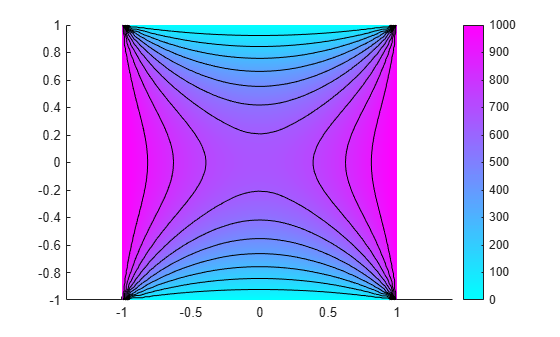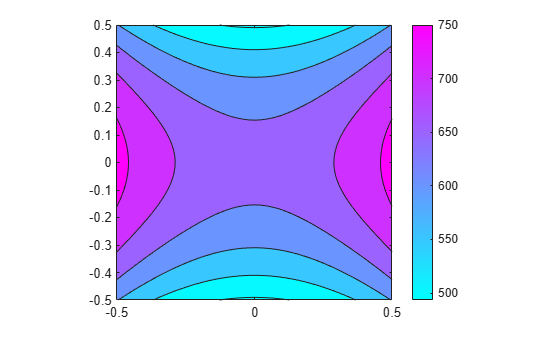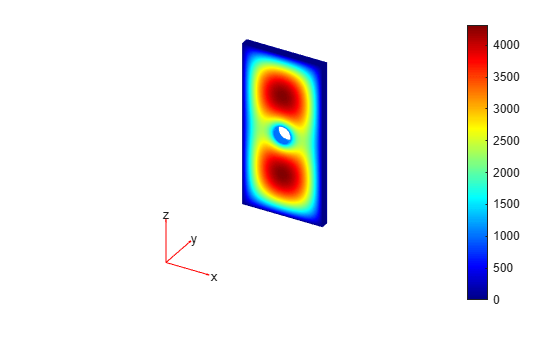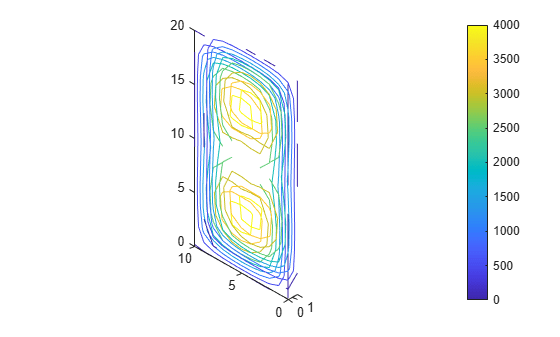interpolateElectricPotential

Interpolate electric potential in electrostatic result at arbitrary spatial locations

Description

example

Vintrp = interpolateElectricPotential(electrostaticresults,xq,yq) returns the interpolated electric potential values at the 2-D points specified in xq and yq.

example

Vintrp = interpolateElectricPotential(electrostaticresults,xq,yq,zq) uses 3-D points specified in xq, yq, and zq.

example

Vintrp = interpolateElectricPotential(electrostaticresults,querypoints) returns the interpolated electric potential values at the points specified in querypoints.

Examples

collapse all

Create an electromagnetic model for electrostatic analysis.

emagmodel = createpde('electromagnetic','electrostatic');

Create a square geometry and include it in the model. Plot the geometry with the edge labels.

R1 = [3,4,-1,1,1,-1,1,1,-1,-1]';
g = decsg(R1, 'R1', ('R1')');
geometryFromEdges(emagmodel,g);
pdegplot(emagmodel,'EdgeLabels','on')
xlim([-1.5 1.5])
axis equalSpecify the vacuum permittivity in the SI system of units.

emagmodel.VacuumPermittivity = 8.8541878128E-12;

Specify the relative permittivity of the material.

electromagneticProperties(emagmodel,'RelativePermittivity',1);

Apply the voltage boundary conditions on the edges of the square.

electromagneticBC(emagmodel,'Voltage',0,'Edge',[1 3]);
electromagneticBC(emagmodel,'Voltage',1000,'Edge',[2 4]);

Specify the charge density for the entire geometry.

electromagneticSource(emagmodel,'ChargeDensity',5E-9);

Generate the mesh.

generateMesh(emagmodel);

Solve the model and plot the electric potential.

R = solve(emagmodel);
pdeplot(emagmodel,'XYData',R.ElectricPotential, ...
'Contour','on')
axis equalInterpolate the resulting electric potential to a grid covering the central portion of the geometry, for x and y from -0.5 to 0.5.

v = linspace(-0.5,0.5,51);
[X,Y] = meshgrid(v);

Vintrp = interpolateElectricPotential(R,X,Y)
Vintrp = 2601×1

602.2959
616.0208
629.0498
641.4049
653.0828
664.0757
674.4209
684.1432
693.2704
701.8026
⋮

Reshape Vintrp and plot the resulting electric potential.

Vintrp = reshape(Vintrp,size(X));

figure
contourf(X,Y,Vintrp)
colormap(cool)
colorbarAlternatively, you can specify the grid by using a matrix of query points.

querypoints = [X(:),Y(:)]';
Vintrp = interpolateElectricPotential(R,querypoints);

Create an electromagnetic model for electrostatic analysis.

emagmodel = createpde('electromagnetic','electrostatic');

Import and plot the geometry representing a plate with a hole.

importGeometry(emagmodel,'PlateHoleSolid.stl');
pdegplot(emagmodel,'FaceLabels','on','FaceAlpha',0.3)Specify the vacuum permittivity in the SI system of units.

emagmodel.VacuumPermittivity = 8.8541878128E-12;

Specify the relative permittivity of the material.

electromagneticProperties(emagmodel,'RelativePermittivity',1);

Specify the charge density for the entire geometry.

electromagneticSource(emagmodel,'ChargeDensity',5E-9);

Apply the voltage boundary conditions on the side faces and the face bordering the hole.

electromagneticBC(emagmodel,'Voltage',0,'Face',3:6);
electromagneticBC(emagmodel,'Voltage',1000,'Face',7);

Generate the mesh.

generateMesh(emagmodel);

Solve the model.

R = solve(emagmodel)
R =
ElectrostaticResults with properties:

ElectricPotential: [4359x1 double]
ElectricField: [1x1 FEStruct]
ElectricFluxDensity: [1x1 FEStruct]
Mesh: [1x1 FEMesh]

Plot the electric potential.

pdeplot3D(emagmodel,'ColorMapData',R.ElectricPotential)Interpolate the resulting electric potential to a grid covering the entire geometry, for x, y, and z.

x = linspace(0,10,11);
y = linspace(0,1,5);
z = linspace(0,20,11);
[X,Y,Z] = meshgrid(x,y,z);

Vintrp = interpolateElectricPotential(R,X,Y,Z);

Reshape Vintrp.

Vintrp = reshape(Vintrp,size(X));

Plot the resulting electric potential as a contour slice plot for two values of the y-coordinate.

figure
contourslice(X,Y,Z,Vintrp,[],[0 1],[])
view([10,10,-10])
axis equal
colorbarInput Arguments

collapse all

Solution of an electrostatic problem, specified as an ElectrostaticResults object. Create electrostaticresults using the solve function.

Example: electrostaticresults = solve(emagmodel)

x-coordinate query points, specified as a real array. interpolateElectricPotential evaluates the electric potential at the 2-D coordinate points [xq(i) yq(i)] or at the 3-D coordinate points [xq(i) yq(i) zq(i)] for every i. Because of this, xq, yq, and (if present) zq must have the same number of entries.

interpolateElectricPotential converts the query points to column vectors xq(:), yq(:), and (if present) zq(:). It returns electric potential values as a column vector of the same size. To ensure that the dimensions of the returned solution are consistent with the dimensions of the original query points, use reshape. For example, use Vintrp = reshape(Vintrp,size(xq)).

Example: xq = [0.5 0.5 0.75 0.75]

Data Types: double

y-coordinate query points, specified as a real array. interpolateElectricPotential evaluates the electric potential at the 2-D coordinate points [xq(i) yq(i)] or at the 3-D coordinate points [xq(i) yq(i) zq(i)] for every i. Because of this, xq, yq, and (if present) zq must have the same number of entries.

interpolateElectricPotential converts the query points to column vectors xq(:), yq(:), and (if present) zq(:). It returns electric potential values as a column vector of the same size. To ensure that the dimensions of the returned solution are consistent with the dimensions of the original query points, use reshape. For example, use Vintrp = reshape(Vintrp,size(yq)).

Example: yq = [1 2 0 0.5]

Data Types: double

z-coordinate query points, specified as a real array. interpolateElectricPotential evaluates the electric potential at the 3-D coordinate points [xq(i) yq(i) zq(i)]. Therefore, xq, yq, and zq must have the same number of entries.

interpolateElectricPotential converts the query points to column vectors xq(:), yq(:), and zq(:). It returns electric potential values as a column vector of the same size. To ensure that the dimensions of the returned solution are consistent with the dimensions of the original query points, use reshape. For example, use Vintrp = reshape(Vintrp,size(zq)).

Example: zq = [1 1 0 1.5]

Data Types: double

Query points, specified as a real matrix with either two rows for 2-D geometry or three rows for 3-D geometry. interpolateElectricPotential evaluates the electric potential at the coordinate points querypoints(:,i) for every i, so each column of querypoints contains exactly one 2-D or 3-D query point.

Example: For a 2-D geometry, querypoints = [0.5 0.5 0.75 0.75; 1 2 0 0.5]

Data Types: double

Output Arguments

collapse all

Electric potential at query points, returned as a vector. For query points that are outside the geometry, Vintrp(i) = NaN.